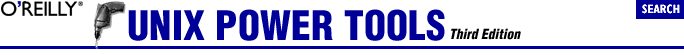home | O'Reilly's CD bookshelfs | FreeBSD | Linux | Cisco | Cisco Exam## 36.21. Quick Reference: expr

### 36.21.1. Syntax

expr arg1 operator arg2 [ operator arg3 ... ]

Arguments and operators must be separated by spaces. In many cases, an argument is an integer, typed literally or represented by a shell variable. There are three types of operators: arithmetic, relational, and logical.

Exit status (Section 35.12) values for expr are 0 if the expression evaluates nonzero and non-null, 1 if the expression evaluates to 0 or null, and 2 if the expression is invalid.

Arithmetic operators
Use these to produce mathematical expressions whose results are printed:

+

-
Subtract arg2 from arg1.

*
Multiply the arguments.

/
Divide arg1 by arg2.

%
Take the remainder when arg1 is divided by arg2 (modulus).

Addition and subtraction are evaluated last, unless they are grouped inside parentheses. The symbols *, (, and ) have meaning to the shell, so they must be escaped (preceded by a backslash or enclosed in quotes).

Relational operators
Use these to compare two arguments. Arguments can also be words, in which case comparisons assume a < z and A < Z. If the comparison statement is true, expr writes 1 to standard output (Section 43.1); if false, it writes 0. The symbols > and < must be escaped.

=
Are the arguments equal?

!=
Are the arguments different?

>
Is arg1 greater than arg2?

>=
Is arg1 greater than or equal to arg2?

<
Is arg1 less than arg2?

<=
Is arg1 less than or equal to arg2?

Logical operators
Use these to compare two arguments. Depending on the values, the result written to standard output can be arg1 (or some portion of it), arg2, or 0. The symbols | and & must be escaped.

|
Logical OR; if arg1 has a nonzero (and non-null) value, the output is arg1; otherwise, the output is arg2.

&
Logical AND; if both arg1 and arg2 have a nonzero (and non-null) value, the output is arg1; otherwise, the output is 0.

:
Sort of like grep (Section 13.1); arg2 is a regular expression (Section 32.4) to search for in arg1. If the arg2 pattern is enclosed in \( \), the output is the portion of arg1 that matches; otherwise, the output is simply the number of characters that match. A pattern match always applies to the beginning of the argument (the ^ symbol (Section 32.5) is assumed by default).